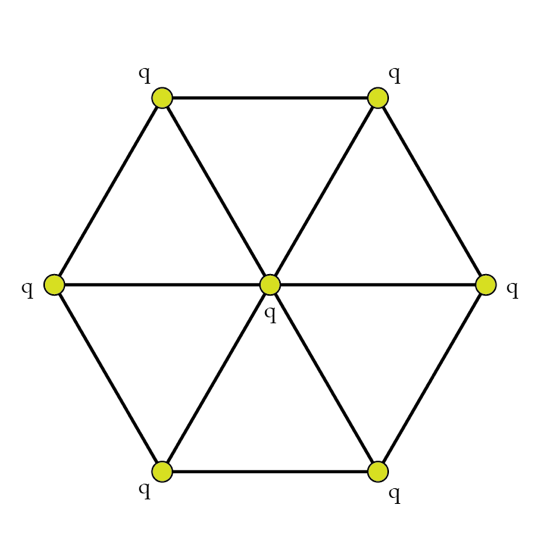# Electrostatic energy and combinatorics

A certain artificial molecule can be modelled as seven identical charges, arranged in a hexagon as shown in the figure. The chemical bonds are modelled as cords of length l. Based on dimensional analysis, the total energy of the system must be of the form $E= A \frac{k q^{2}}{l}, \quad k=\frac{1}{4\pi \epsilon_{0}}$ where A is some numerical coefficient. Determine A.×

Problem Loading...

Note Loading...

Set Loading...